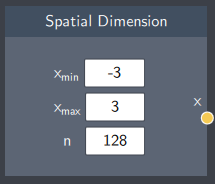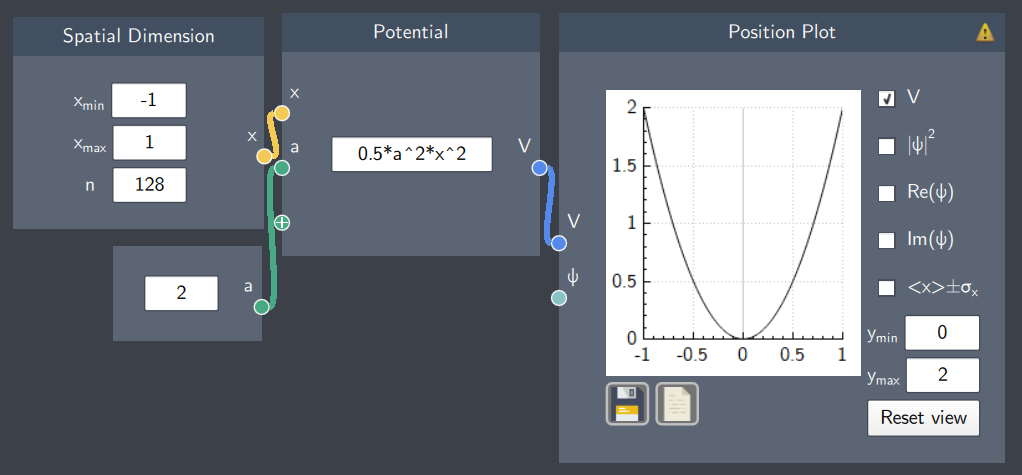# Spatial Dimension

## Description

In this node, the spatial dimension of your system is defined. It represents the bounds and the resolution of the system along the $x$-axis.## Input

The node does not require any input from other nodes.

## Content

The Spatial Dimension node consists of  3 content fields that are used to generate the discrete spatial dimension. $x_{min}$ and $x_{max}$ represent the lower and upper bound of the spatial dimension respectively and $n$ is the number of points within this range.

Note:

1. The range of $x$ represents the bounds for the simulation. As far as the simulation is concerned, space is one-dimensional and lies only between $x_{min}$ and $x_{max}$.
2. The value of $n$ by default is set to 128 points but if necessary, it can be changed to a higher value. The value of $n$ should be power of 2.

## Output

• Spatial Dimension ($x$): It outputs $n$ values of $x$ within a specified range based on the content fields.

## Example

The spatial dimension has been defined from -1 to 1 along the $x$-axis and divided by 128 points. The output is used to define the bounds of a harmonic oscillator potential as shown in the plot.